Learning Library

Find the Missing Numbers: Reverse Addition & Subtraction

Your kid will play digit detective as she solves the case of the missing number.

Click on a worksheet in the set below to see more info or download the PDF.Find the Missing Numbers: Reverse Addition & Subtraction #1

Kids practice reverse two-digit addition and subtraction to find the missing numbers in this 2nd grade math worksheet.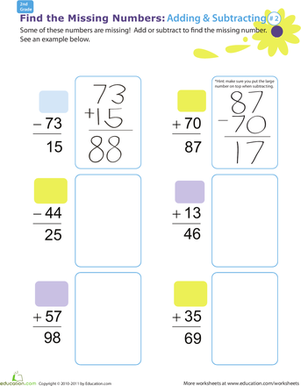Find the Missing Numbers: Reverse Addition & Subtraction #2

Introduce your child to basic algebra with this 2nd grade math worksheet. Kids practice reverse addition or subtraction to find the missing numbers.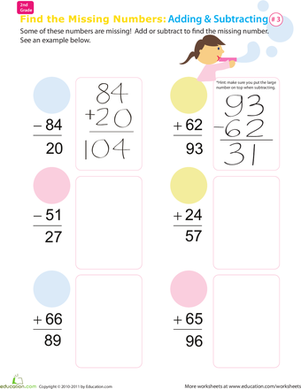Find the Missing Numbers: Reverse Addition & Subtraction #3

Kids practice reverse addition and subtraction to find the missing numbers in this fun 2nd grade math worksheet.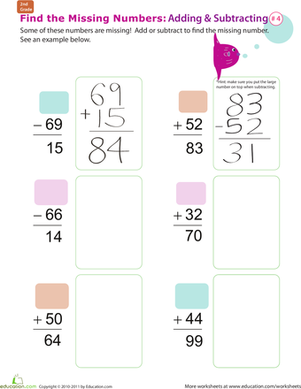Find the Missing Numbers: Reverse Addition & Subtraction #4

Kids get a taste of basic algebra in this 2nd grade math worksheet. Kids flip the equations and reverse the operations in order to find the missing number.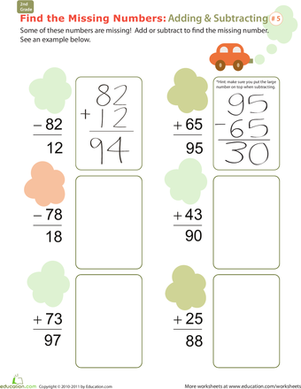Find the Missing Numbers: Reverse Addition & Subtraction #5

Kids get an intro to basic algebra by practicing reverse addition and subtraction to find the missing numbers in this challenging 2nd grade math worksheet.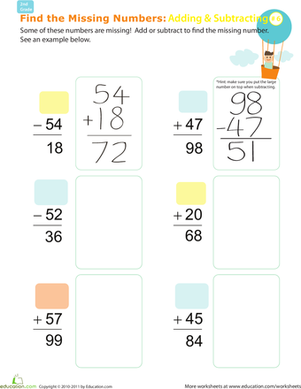Find the Missing Numbers: Reverse Addition & Subtraction #6

Kids practice reverse addition and subtraction to find the missing numbers in this challenging 2nd grade math worksheet.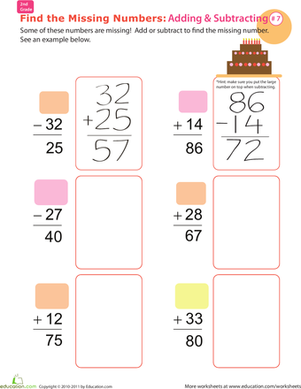Find the Missing Numbers: Reverse Addition & Subtraction #7

Kids try their hand at reverse addition and subtraction in order to find the numbers missing from each equation in this 2nd grade math worksheet.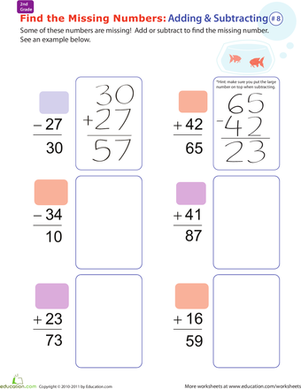Find the Missing Numbers: Reverse Addition & Subtraction #8

Introduce your child to basic algebra with this 2nd grade math worksheet in which he'll practice reverse addition and subtraction to find the missing numbers.

Want to download this whole set as a single PDF?

Create new collection

0

New Collection>

0 items

What could we do to improve Education.com?

Please note: Use the Contact Us link at the bottom of our website for account-specific questions or issues.

What would make you love Education.com?